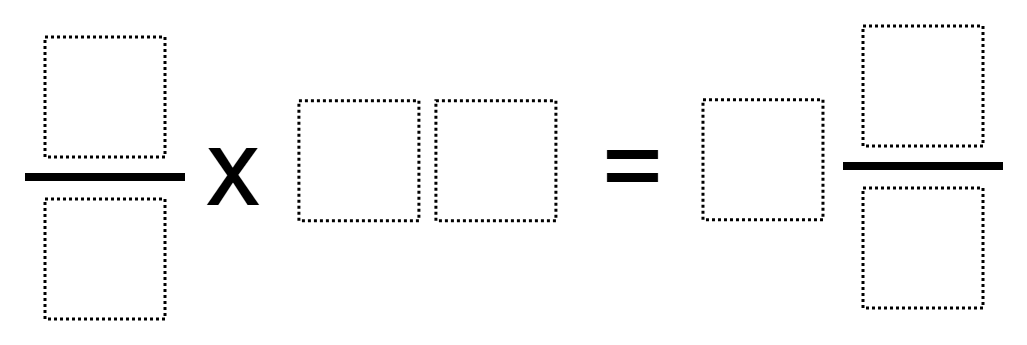# Multiplying Fraction by a 2-Digit Whole Number

Directions: Using the digits 0 through 9 at most one time, place a digit in each box to make a true equation.### Hint

How can you make sure that your product is a mixed number?
Are there some two-digit numbers that don’t divide equally into groups?
How can you rename fractions so that you don’t use the same digit more than once?

There are multiple solutions. One is 2/4 x 17 = 8 3/6.

Source: Ellen Metzger

## Equivalent Expressions with Fractions

Directions: Using the digits 0 to 9 at most one time each and choosing either …

1.2.3.4.